How Cheenta works to ensure student success?
Explore the Back-Story

# Test of Mathematics Solution Subjective 72 - Polynomial Problem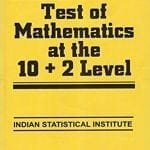This is a Test of Mathematics Solution Subjective 72 (from ISI Entrance). The book, Test of Mathematics at 10+2 Level is Published by East West Press. This problem book is indispensable for the preparation of I.S.I. B.Stat and B.Math Entrance.

Also visit: I.S.I. & C.M.I. Entrance Course of Cheenta

## Problem

Ifare the roots of the equation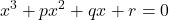, find the equation whose roots are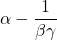,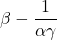,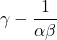.

## Solutionare roots of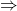=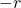,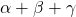=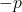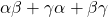=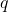Now,+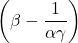+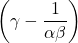=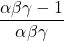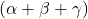= -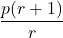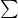=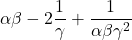=- 2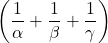+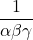+=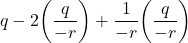=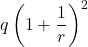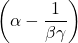++=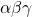- 3 +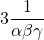-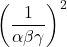= -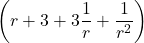So the equation whose roots are,,isThis is a Test of Mathematics Solution Subjective 72 (from ISI Entrance). The book, Test of Mathematics at 10+2 Level is Published by East West Press. This problem book is indispensable for the preparation of I.S.I. B.Stat and B.Math Entrance.

Also visit: I.S.I. & C.M.I. Entrance Course of Cheenta

## Problem

Ifare the roots of the equation, find the equation whose roots are,,.

## Solutionare roots of=,==Now,++== -==- 2++==++=- 3 +-= -So the equation whose roots are,,is### Knowledge Partner